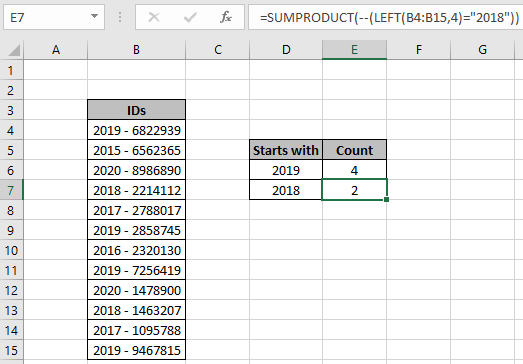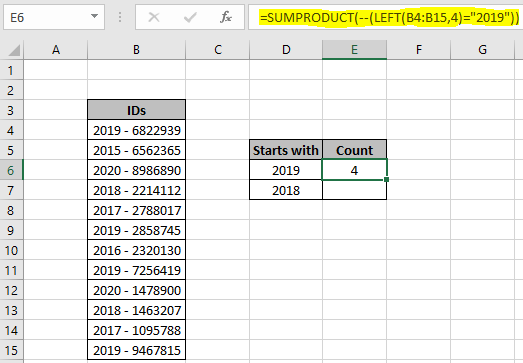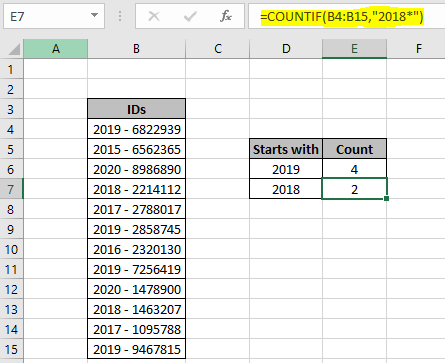# How to count numbers that begin with specific value ExcelIn this article, we will learn How to count numbers that begin with a given specific value Excel.

Scenario :

At instances when working with datasets. Sometimes we need to just get how many cells in the list which begins with a particular number. For Example finding the count of scores (above 10) in Exam where the student scored marks from 70 to 79 out of 100. For the above example you can use the double criteria one is number >= 70 & number <=79. Let's understand how we can solve this kind of problem using only one criteria using the explained formula in Excel.

How to solve the problem?

For this, we will be using the SUMPRODUCT function and wildcard ( * ). SUMPRODUCT function counts the matched numbers. Here the criteria is the number must start with a particular given value. String can have any datatype like date, time, names or numbers. ( * ) character matches any number of uncertain characters in the string. LEFT function will extract the starting number from the string and match it with the given value in Excel.

Generic formula:

 =SUMPRODUCT(--(LEFT(range,n)="num")

range : list of values to match

num : given specific number

n : length of num value

Example :

All of these might be confusing to understand. Let's understand this formula using it in an example. Here we have a list of Ids having different year values attached as prefix. We need to find the count of IDs which start with 2019 year.

Use the formula:

 =SUMPRODUCT(--(LEFT(B4:B15,4)="2019"))

Explanation :

1. LEFT function extracts the first 4 characters from the list of IDs and matches it with the year value 2019.
2. The above process will create an array of TRUE and FALSE values. True value corresponding to the matched criteria.
3. SUMPRODUCT counts the number of TRUE values in the array and returns the count.There are 4 Ids in the list (B4:B15) which lies in 2019. You can get the count of IDS having any year value from the Id list.

Now we can perform this function using the COUNTIF function. COUNTIF function returns the count of numbers using the asterisk wildcard. ( * ) wildcard matches any number of uncertain characters in length.

Use the formula:

 =COUNTIF(B4:B15,"2018*"))

B3:B14 : range to match with

* : wildcard use to match any suffix with 2018.As you can see there are 2 Ids which do not lay in 2018. If you have more than one criteria to match then use the COUNTIFS function in Excel. You can also extract the count of numbers which ends with a given value using wildcard here.

Use the SUMPRODUCT function to count rows in the list. Learn more about Countif with SUMPRODUCT in Excel here.

Here are some observational notes shown below.

Notes:

1. The formula works with numbers, text, date values, etc.
2. Use the same data type to match criteria in criteria list like name in names list, date in date values.
3. Lists as criteria ranges must be of same length, or the formula returns #VALUE! error.
4. Operators like equals to ( = ), less than equal to ( <= ), greater than ( > ) or not equals to ( <> ) can be used in criteria arguments, with numbers list only.
5. For example matching time values which have hour value 6. So you can use "06:*:*". Each ( * ) asterisk character is used for the uncertain minutes and seconds.

Hope this article about How to count numbers that begin with specific value Excel is explanatory. Find more articles on Counting values in the table using formulas here. If you liked our blogs, share it with your friends on Facebook. And also you can follow us on Twitter and Facebook. We would love to hear from you, do let us know how we can improve, complement or innovate our work and make it better for you. Write us at info@exceltip.com

Related Articles :

How to use the COUNTIF Function in Excel : Count values with conditions using this amazing function. You don't need to filter your data to count specific values. Countif function is essential to prepare your dashboard.

How to use the SUMPRODUCT function in Excel: Returns the SUM after multiplication of values in multiple arrays in excel.

COUNTIFS with Dynamic Criteria Range : Count cells selecting the criteria from the list of options in criteria cell in Excel using data validation tool.

COUNTIFS Two Criteria Match : multiple criteria match in different lists in table using the COUNTIFS function in Excel

COUNTIFS With OR For Multiple Criteria : match two or more names in the same list using the OR criteria applied on the list in Excel.

How to Use Countif in VBA in Microsoft Excel : Count cells with criteria using Visual Basic for Applications code in Excel macros.

How to use wildcards in excel : Count cells matching phrases in text lists using the wildcards ( * , ? , ~ )in excel

Popular Articles:

How to use the IF Function in Excel : The IF statement in Excel checks the condition and returns a specific value if the condition is TRUE or returns another specific value if FALSE.

How to use the VLOOKUP Function in Exceln : This is one of the most used and popular functions of excel that is used to lookup value from different ranges and sheets.

How to Use SUMIF Function in Excel : This is another dashboard essential function. This helps you sum up values on specific conditions.